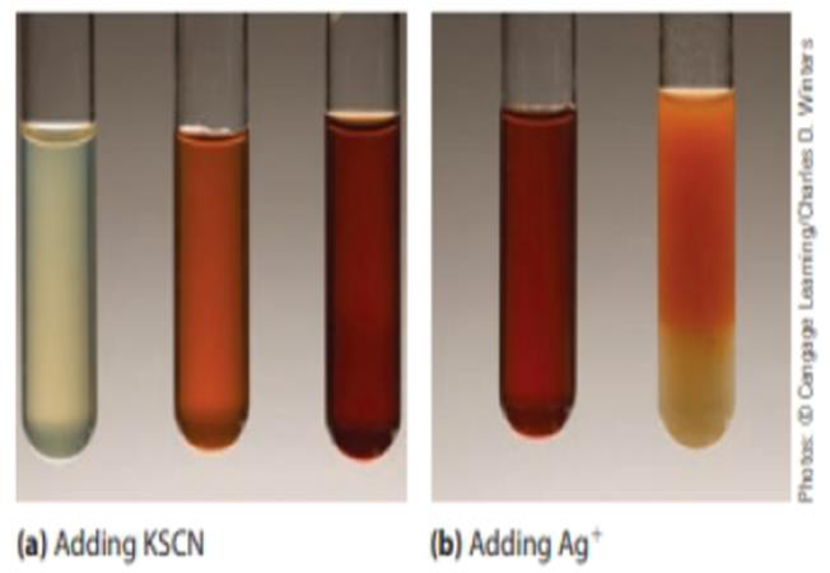Chapter 15, Problem 67IL

Chapter
Section
Textbook Problem

The photographs below (a) show what occurs when a solution of iron(III) nitrate is treated with a few drops of aqueous potassium thiocyanate. The nearly colorless iron(III) ion is converted to a red [Fe(H2O)5SCN)2+ ion. (This is a classic test for the presence of iron(III) ions in solution.)[Fe(H2O)6]3+(aq) + SCN−(aq) ⇄ [Fe(H2O)5SCN]2+(aq) + H2O(ℓ)(a) As more KSCN is added to the solution, the color becomes even more red. Explain this observation. (b) Silver ions form a white precipitate with SCN− ions. What would you observe on adding a few drops of aqueous silver nitrate to a red solution of [Fe(H2O)5 SCN]+ ions? Explain your observation.

(a)

Interpretation Introduction

Interpretation:

The reason for increase in red colour as more KSCN is added in the reaction of iron nitrate with potassium thiocyanate has to be explained.

Concept Introduction:

Le Chatelier’s principle: If equilibrium is disturbed by changing conditions, the system will moves the equilibrium to reverse the change.

Factor’s that effect chemical equilibria:

• Concentration – Equilibrium will be affected by changing the concentration of reactant or product. If we increase the concentration of reactant system will try to reverse the change by favouring forward reaction and thus increase the concentration of products. Likewise adding products increase yield of reactants.
• Temperature – When the temperature increases equilibrium will shift in the endothermic direction, in the direction that absorbs heat. When the temperature decreases equilibrium will shift in the exothermic direction, in the direction that releases heat.
• Pressure – If the reaction consists of only liquid and solid reactants and products, pressure has no effect in the equilibrium.

In gas reactions if the number of moles has no change then there will be no effect by pressure on equilibrium.

If pressure increases then equilibrium will shift to the direction having less number of molecules and if pressure decreases system will shift to the direction having more number of molecules.

Test for Fe+3solution: The presence of Fe+3 can be confirmed by using the solution of potassium thiocyanate. Reaction with potassium thiocyanate gives a blood red colour solution due to the formation of [Fe(H2O)5SCN]+ ions.

Explanation

Given:

The classic test for identifying the presence of Fe+3 ions,

[Fe(H2O)6]+3(aq)+SCN-(aq)[Fe(H2O)5SCN]+2(aq)

(b)

Interpretation Introduction

Interpretation:

The observation on adding a few drops of aqueous silver nitrate to a red solution of [Fe(H2O)5SCN]+ ions has to be explained.

Concept Introduction:

Le Chatelier’s principle: If an equilibrium is disturbed by changing conditions, the system will moves the equilibrium to reverse the change.

Factor’s that effect chemical equilibria:

• Concentration – Equilibrium will be affected by changing the concentration of reactant or product. If we increase the concentration of reactant system will try to reverse the change by favouring forward reaction and thus increase the concentration of products. Likewise adding products increase yield of reactants.
• Temperature – When the temperature increases equilibrium will shift in the endothermic direction, in the direction that absorbs heat. When the temperature decreases equilibrium will shift in the exothermic direction, in the direction that releases heat.
• Pressure – If the reaction consists of only liquid and solid reactants and products, pressure has no effect in the equilibrium.

In gas reactions if the number of moles has no change then there will be no effect by pressure on equilibrium.

If pressure increases then equilibrium will shift to the direction having less number of molecules and if pressure decreases system will shift to the direction having more number of molecules.

Test for Fe+3solution: The presence of Fe+3 can be confirmed by using the solution of potassium thiocyanate. Reaction with potassium thiocyanate gives a blood red colour solution due to the formation of [Fe(H2O)5SCN]+ ions.

Still sussing out bartleby?

Check out a sample textbook solution.

See a sample solution

The Solution to Your Study Problems

Bartleby provides explanations to thousands of textbook problems written by our experts, many with advanced degrees!

Get Started

Athletes should avoid frequent between-meal snacks. T F

Nutrition: Concepts and Controversies - Standalone book (MindTap Course List)

What are derived units?

An Introduction to Physical Science

What are genomes?

Human Heredity: Principles and Issues (MindTap Course List)